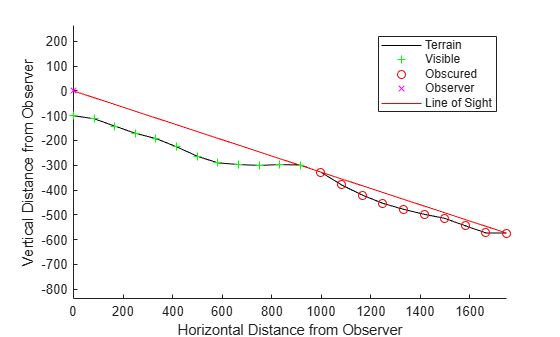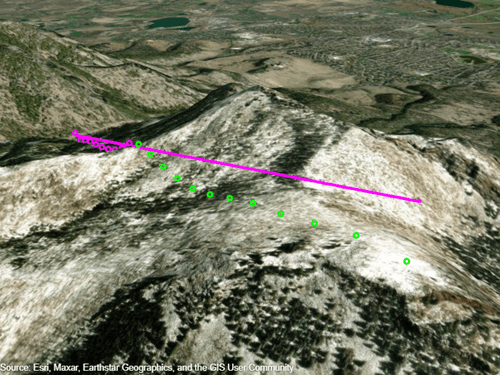# los2

Line-of-sight visibility between two points on or above terrain

## Syntax

``vis = los2(Z,R,lat1,lon1,lat2,lon2)``
``vis = los2(Z,R,lat1,lon1,lat2,lon2,h1)``
``vis = los2(Z,R,lat1,lon1,lat2,lon2,h1,h2)``
``vis = los2(Z,R,lat1,lon1,lat2,lon2,h1,h2,h1ref)``
``vis = los2(Z,R,lat1,lon1,lat2,lon2,h1,h2,h1ref,h2ref)``
``vis = los2(Z,R,lat1,lon1,lat2,lon2,h1,h2,h1ref,h2ref,rad)``
``vis = los2(Z,R,lat1,lon1,lat2,lon2,h1,h2,h1ref,h2ref,rad,effectiveRad)``
``[vis,visprofile,dist,h,lattrk,lontrk] = los2(___)``
``los2(___)``
``[___] = los2``

## Description

### Specify Coordinates and Heights

````vis = los2(Z,R,lat1,lon1,lat2,lon2)` determines whether the point specified by `lat1` and `lon1` has line-of-sight visibility with the point specified by `lat2` and `lon2`. Specify spatially referenced terrain data, in meters, using `Z` and `R`. This function assumes that the Earth is a sphere.```

example

````vis = los2(Z,R,lat1,lon1,lat2,lon2,h1)` specifies the height, in meters, of the first point.```
````vis = los2(Z,R,lat1,lon1,lat2,lon2,h1,h2)` specifies the height, in meters, of the second point.```
````vis = los2(Z,R,lat1,lon1,lat2,lon2,h1,h2,h1ref)` references the height of the first point to either the terrain (ground level) or the sphere (mean sea level).```
````vis = los2(Z,R,lat1,lon1,lat2,lon2,h1,h2,h1ref,h2ref)` references the height of the second point to either the terrain (ground level) or the sphere (mean sea level).```

### Specify Reference Sphere

````vis = los2(Z,R,lat1,lon1,lat2,lon2,h1,h2,h1ref,h2ref,rad)` specifies the radius in meters of the reference sphere. This syntax is useful for finding line-of-sight visibility for planetary bodies other than Earth.```
````vis = los2(Z,R,lat1,lon1,lat2,lon2,h1,h2,h1ref,h2ref,rad,effectiveRad)` specifies a larger radius for the propagation of line-of-sight paths. You can use this syntax to account for the curvature of signal paths due to refraction in the atmosphere.```

### Query Intermediate Points

example

````[vis,visprofile,dist,h,lattrk,lontrk] = los2(___)` determines line-of-sight visibility for intermediate points between the first and second points, using any combination of input arguments from the previous syntaxes. For each intermediate point, the function returns the visibility in `visprofile`, the distance from the first point in `dist`, the height in `h`, the latitude in `lattrk`, and the longitude in `lontrk`. The number of intermediate points depends on the resolution of the terrain data.```

### Display and Interactively Select Coordinates

example

````los2(___)` displays the visibility profile between the two points in a new figure on Cartesian axes. The figure illustrates the terrain, the observer, the line of sight, the visible points, and the obstructed points.```
````[___] = los2` enables you to interactively select two points on the current `axesm`-based map and then determines whether the points have line-of-sight visibility, returning any combination of output arguments from previous syntaxes. If the current object on the map is a surface, then the function uses the z-coordinate data (the `ZData` property) as the terrain elevation data. Otherwise, the function uses z-coordinate data from the first surface it finds on the map. If the surface does not have z-coordinate data, then the function uses the color data (the `CData` property), instead.```

## Examples

collapse all

Determine whether two points have line-of-sight visibility. Then, display the visibility profile.

Read terrain data for an area around South Boulder Peak into the workspace as an array and a reference object. Prepare the data for analysis by specifying the output type as `"double"`.

`[Z,R] = readgeoraster("n39_w106_3arc_v2.dt1","OutputType","double");`

Specify the coordinates of South Boulder Peak and a nearby point.

```lat1 = 39.95384; lon1 = -105.29916; lat2 = 39.96955; lon2 = -105.29867;```

Determine whether the nearby point is visible from a location `100` meters above the peak. The result indicates that the points do not have line-of-sight visibility.

```h1 = 100; vis = los2(Z,R,lat1,lon1,lat2,lon2,h1)```
```vis = logical 0 ```

Display the visibility profile by omitting the output argument.

`los2(Z,R,lat1,lon1,lat2,lon2,h1)`To view a visibility profile in 3-D, calculate the profile using the `los2` function and display the profile using the `geoglobe` and `geoplot3` functions.

Read terrain data for an area around South Boulder Peak into the workspace as an array and a reference object. Prepare the data for analysis by specifying the output type as `"double"`.

`[Z,R] = readgeoraster("n39_w106_3arc_v2.dt1","OutputType","double");`

Specify the coordinates of South Boulder Peak and a nearby point.

```lat1 = 39.95384; lon1 = -105.29916; lat2 = 39.96955; lon2 = -105.29867;```

Determine whether the nearby point is visible from a location `100` meters above the peak. The result indicates that the points do not have line-of-sight visibility.

```h1 = 100; vis = los2(Z,R,lat1,lon1,lat2,lon2,h1)```
```vis = logical 0 ```

Calculate visibility information for the intermediate points. The outputs `lattrk` and `lontrk` contain the latitude and longitude coordinates of the intermediate points. The output `visprofile` indicates whether the intermediate points are visible from South Boulder Peak.

`[~,visprofile,~,~,lattrk,lontrk] = los2(Z,R,lat1,lon1,lat2,lon2,100);`

Create a geographic globe that uses custom terrain for the area around South Boulder Peak.

```addCustomTerrain("southboulder","n39_w106_3arc_v2.dt1") uif = uifigure; g = geoglobe(uif,"Terrain","southboulder");```

Plot the line of sight from South Boulder Peak to the nearby point. Use a magenta line to indicate the point is not visible from above the peak.

```geoplot3(g,[lat1 lat2],[lon1 lon2],[h1 0],"m","LineWidth",2, ... "HeightReference","terrain")```

Plot the intermediate points. Use magenta markers for points that are not visible and green markers for points that are visible. Plot each value `10` meters above the terrain so the markers are not obscured by the terrain.

```hold(g,"on") geoplot3(g,lattrk(~visprofile),lontrk(~visprofile),10, ... "mo","MarkerSize",2,"LineWidth",2,"HeightReference","terrain") geoplot3(g,lattrk(visprofile),lontrk(visprofile),10,"go", ... "MarkerSize",2,"LineWidth",2,"HeightReference","terrain")```

Adjust the view by setting the camera position, pitch, roll, and heading.

```campos(g,39.9519,-105.3039,2.9424e+03) campitch(g,-31.7850) camroll(g,0.3603) camheading(g,36.5618)```Close the figure containing the geographic globe and remove the custom terrain.

```close(uif) removeCustomTerrain("southboulder")```

## Input Arguments

collapse all

Elevation data grid, in meters, specified as an m-by-n array.

Data Types: `single` | `double`

Spatial reference for `Z`, specified as a `GeographicCellsReference` or `GeographicPostingsReference` object. The `RasterSize` property of `R` must be consistent with `size(Z)`.

Latitude of the first point in degrees, specified as a scalar or vector.

The sizes of `lat1`, `lon1`, `lat2`, and `lon2` must match.

Data Types: `single` | `double`

Longitude of the first point, in degrees, specified as a scalar or vector.

The sizes of `lat1`, `lon1`, `lat2`, and `lon2` must match.

Data Types: `single` | `double`

Latitude of the second point, in degrees, specified as a scalar or vector.

The sizes of `lat1`, `lon1`, `lat2`, and `lon2` must match.

Data Types: `single` | `double`

Longitude of the second point, in degrees, specified as a scalar or vector.

The sizes of `lat1`, `lon1`, `lat2`, and `lon2` must match.

Data Types: `single` | `double`

Height of the first point, in meters, specified as a scalar or vector.

The size of `h1` must match the size of `lat1` and `lon1`.

Data Types: `single` | `double`

Height of the second point, in meters, specified as a scalar or vector.

The size of `h2` must match the size of `lat2` and `lon2`.

Data Types: `single` | `double`

Height reference for the first point, specified as one of these options:

• `"AGL"` — Reference `h1` to the terrain (ground level).

• `"MSL"` — Reference `h1` to the sphere (mean sea level).

Data Types: `char` | `string`

Height reference for the second point, specified as one of these options:

• `"AGL"` — Reference `h2` to the terrain (ground level).

• `"MSL"` — Reference `h2` to the sphere (mean sea level).

Data Types: `char` | `string`

Radius of the reference sphere, in meters, specified as a positive scalar.

Data Types: `single` | `double`

Effective radius of the reference sphere, in meters, specified as a positive scalar.

This argument enables you to account for the curvature of signal paths due to refraction in the atmosphere. For example, you can treat radio propagation in the atmosphere as straight-line propagation on a sphere with 4/3 the radius of the Earth by specifying `rad` as `6371000` and `effectiveRad` as `4/3*6371000`.

To calculate line-of-sight visibility for a flat Earth, specify this argument as `Inf`.

If you do not specify this argument, then the function uses the value of `rad`.

Data Types: `single` | `double`

## Output Arguments

collapse all

Indicator for visibility between the first and second points, returned as a logical scalar or a logical array. The size of `vis` matches the size of `lat1`.

• A logical `1` (`true`) indicates that the first point has line-of-sight visibility with the corresponding second point.

• A logical `0` (`false`) indicates that the line of sight between the first point and corresponding second point is obscured by terrain.

Indicator for visibility between the first point and intermediate points, returned as a logical vector or a cell array of logical vectors.

• A logical `1` (`true`) indicates that the first point has line-of-sight visibility with that intermediate point.

• A logical `0` (`false`) indicates that the line of sight between the first point and that intermediate point is obscured by terrain.

The function returns a logical vector when `lat1`, `lon1`, `lat2`, and `lon2` are scalars. The function returns a cell array of logical vectors when `lat1`, `lon1`, `lat2`, and `lon2` are vectors. The number of cells within the cell array matches the size of `lat1`.

Distance from the first point to the intermediate point, in meters, returned as a numeric vector or a cell array of numeric vectors.

The function returns a numeric vector when `lat1`, `lon1`, `lat2`, and `lon2` are scalars. The function returns a cell array of numeric vectors when `lat1`, `lon1`, `lat2`, and `lon2` are vectors. The number of cells within the cell array matches the size of `lat1`.

Height of intermediate points, in meters, returned as a numeric vector or a cell array of numeric vectors.

The function returns a numeric vector when `lat1`, `lon1`, `lat2`, and `lon2` are scalars. The function returns a cell array of numeric vectors when `lat1`, `lon1`, `lat2`, and `lon2` are vectors. The number of cells within the cell array matches the size of `lat1`.

Latitude of intermediate points, in degrees, returned as a numeric vector or a cell array of numeric vectors.

The function returns a numeric vector when `lat1`, `lon1`, `lat2`, and `lon2` are scalars. The function returns a cell array of numeric vectors when `lat1`, `lon1`, `lat2`, and `lon2` are vectors. The number of cells within the cell array matches the size of `lat1`.

Longitude of intermediate points, in degrees, returned as a numeric vector or a cell array of numeric vectors.

The function returns a numeric vector when `lat1`, `lon1`, `lat2`, and `lon2` are scalars. The function returns a cell array of numeric vectors when `lat1`, `lon1`, `lat2`, and `lon2` are vectors. The number of cells within the cell array matches the size of `lat1`.

## Version History

Introduced before R2006a

expand all